#### Logistic RegressionAnalytics Vidhya

Example: flipping a coin one time. The probability of getting a head = 𝑝 ( ℎ𝑒𝑎𝑑 )=1/2 Introduction Logistic regression is a supervised learning technique , which is basically a probabilistic class...#### Logistic RegressionTowards Data Science

Logistic Regression is one of the fundamental models used in Machine Learning. It is a classification technique and is best suited for predicting the categorical response variable. While linear…

Read more at Towards Data Science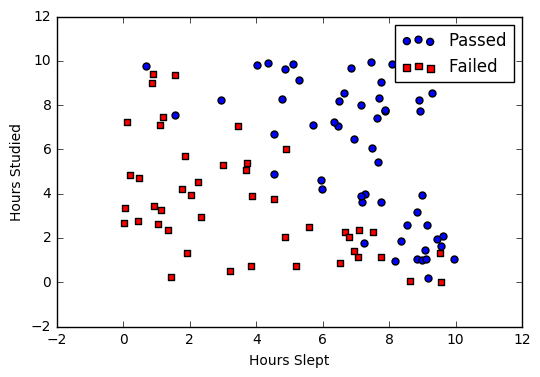#### Logistic RegressionMachine Learning Glossary

Logistic Regression Introduction Comparison to linear regression Types of logistic regression Binary logistic regression Sigmoid activation Decision boundary Making predictions Cost function Gradient ...

Read more at Machine Learning Glossary#### Data Science : Logistic RegressionAnalytics Vidhya

Logistic Regression is supervised machine learning algorithm, used for binary class or multi class classification(also called one-vs-all Classification). This is one of the famous classification…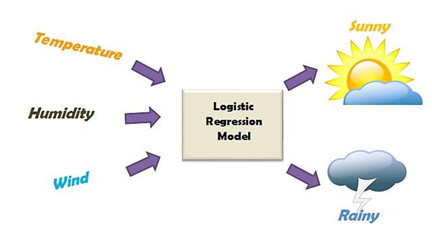#### Logistic Regression.Analytics Vidhya

Logistic regression is a classification algorithm used to assign observations to a discrete set of classes. Unlike linear regression which outputs continuous number values, logistic regression…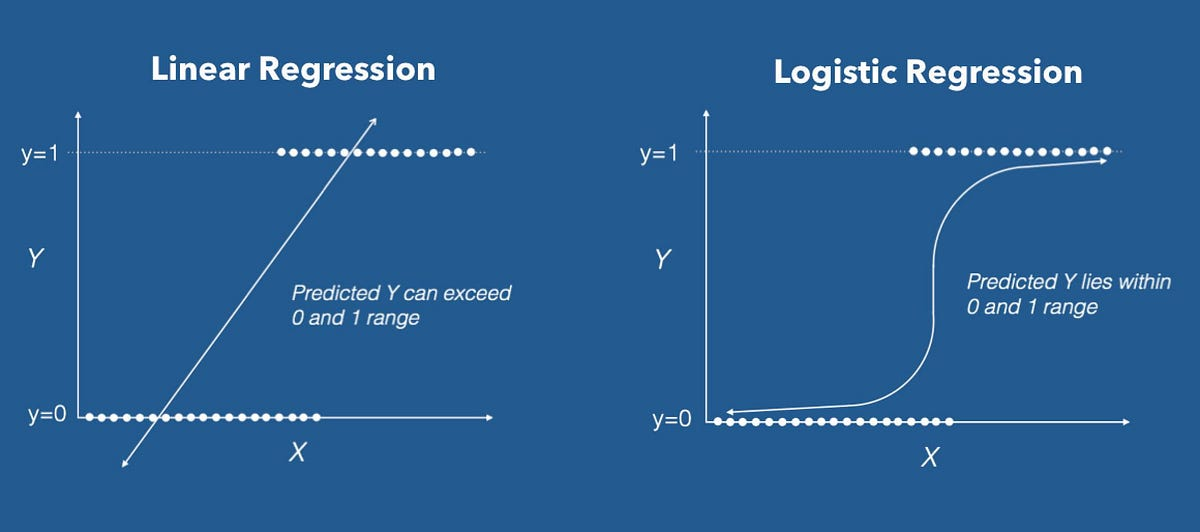#### Logistic Regression!!!Analytics Vidhya

Now, to predict whether a user will purchase the product or not, one needs to find out the relationship between Age and Estimated Salary. Here User ID and Gender are not important factors for finding…...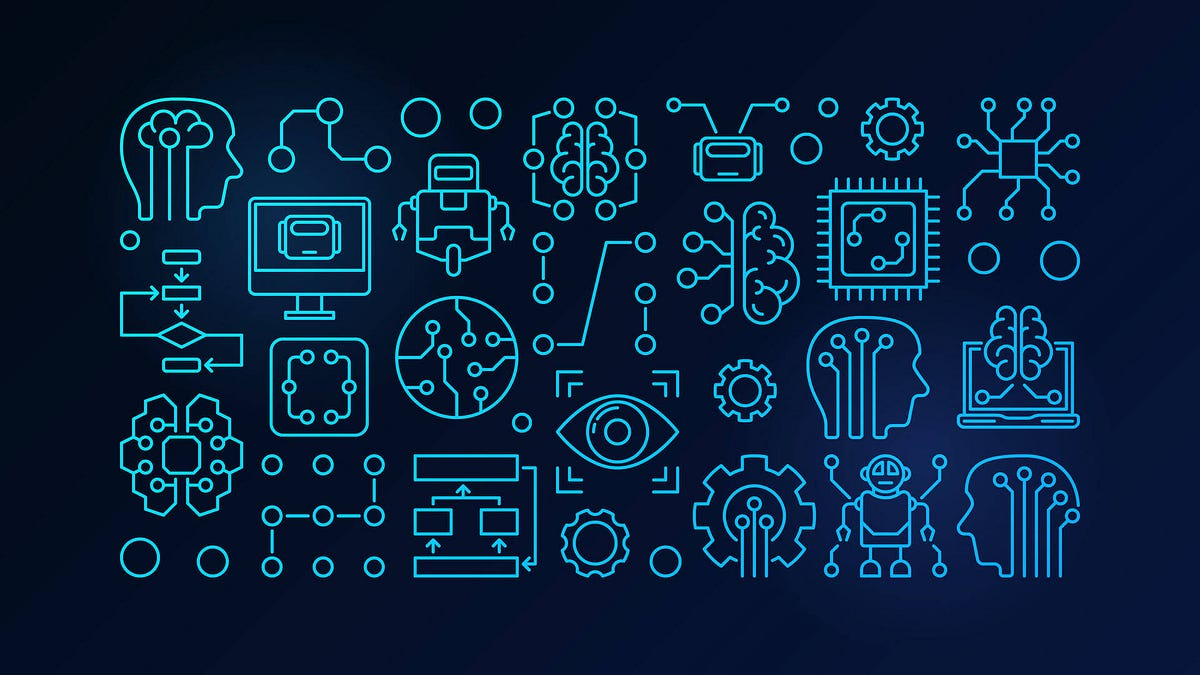#### Chapter 5 — Logistic RegressionAnalytics Vidhya

In previous blogs we used the Linear Regression technique to create models. But Linear Regression technique is unbounded, it has infinite possibilities and can be used only when the response variable…...#### Logistic RegressionChristophm Interpretable Machine Learning Book

Logistic regression models the probabilities for classification problems with two possible outcomes. It’s an extension of the linear regression model for classification problems. The linear regression...

Read more at Christophm Interpretable Machine Learning Book#### Machine Learning 102: Logistic RegressionTowards Data Science

In a previous article I explored linear regression — the foundation of all other advanced models used in machine learning and data science. Linear regression models continuous dependent variables…

Read more at Towards Data Science#### Basics and Beyond: Logistic RegressionAnalytics Vidhya

This post will walk you through logistic regression from the very basics. In order to master machine learning it is imperative to have the basics very clear. It may seem exhausting at first but once…#### Inside: Logistic RegressionTowards Data Science

A Comprehensive blog on Logistic Regression with explanation consisting of Math behind it and implementing it from scratch through code, along with R^2 and p-value evaluations.

Read more at Towards Data Science#### Logistic Regression - OverviewLearn Data Science

Logistic Regression - Overview What are the odds that an event will happen? Answering yes/no questions. Often we have to resolve questions with binary or yes/no outcomes. For example: Does a patient h...

Read more at Learn Data Science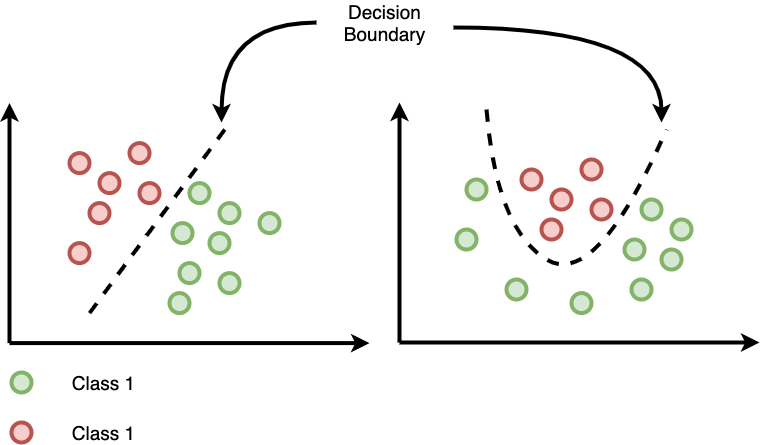#### Logistic Regression theoryAnalytics Vidhya

Logistic regression is a Classification algorithm that will draw a boundary to differentiate between 2 or more classes, the boundary can be linear or non-linear. The starting logic is same as in the…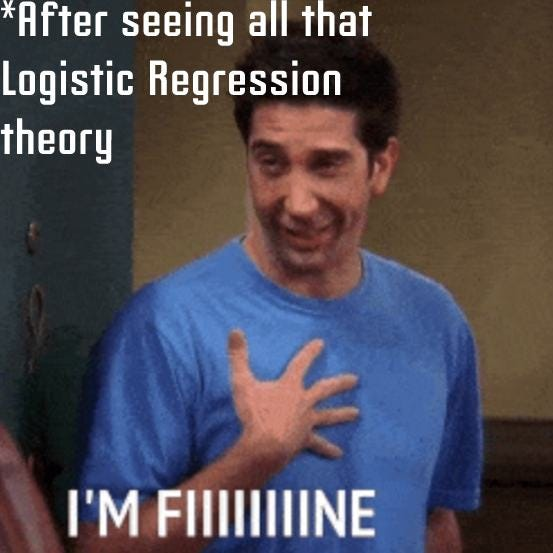#### Logistic Regression : All You wanna KnowAnalytics Vidhya

Logistic Regression is used to model probability of a certain class so that it can be assigned value of 0 or 1. It is a statistical and supervised learning model. It is a go-to method for binary…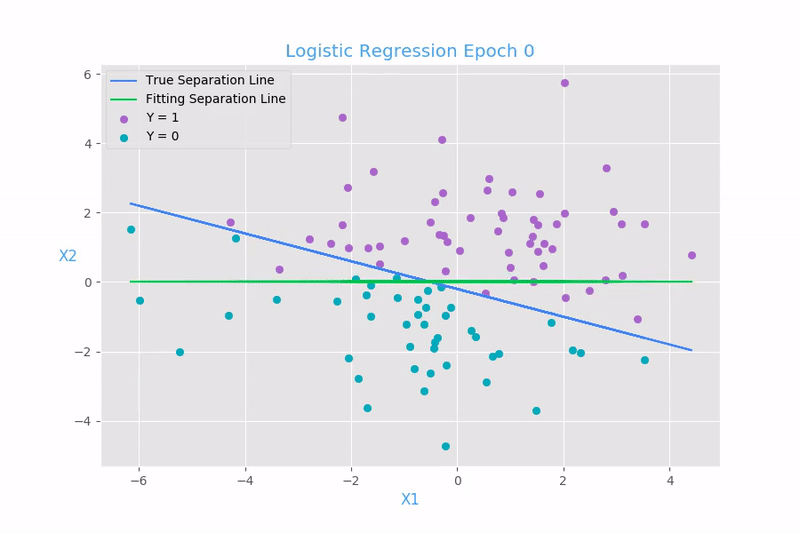Towards Data Science

Unlike other Algorithms, Logistic Data Science Machine learning NLP natural learning artificial intelligence data science Log data algorithms

Read more at Towards Data Science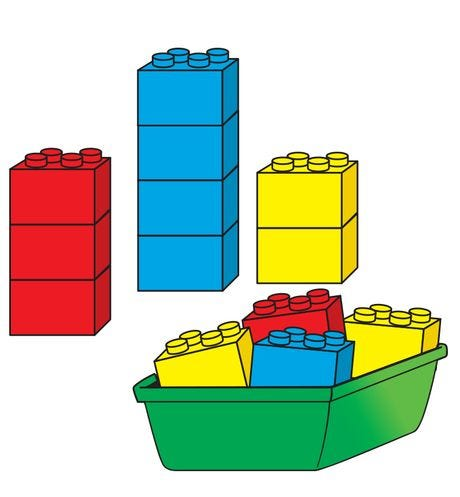#### Classification with Logistic Regression.Analytics Vidhya

The classification is the process of determining which set of categories, also known as sub population a new observation or a new instance belongs to. Before determining for a new instance, a model…#### Binary Logistic RegressionTowards Data Science

Logistic Regression is a classification algorithm which is used when we want to predict a categorical variable (Yes/No, Pass/Fail) based on a set of independent variable(s). In the Logistic…

Read more at Towards Data Science#### Logistic regression: the basicsTowards Data Science

Logistic regression is a technique for modelling the probability of an event. Just like linear regression, it helps you understand the relationship between one or more variables and a target…

Read more at Towards Data Science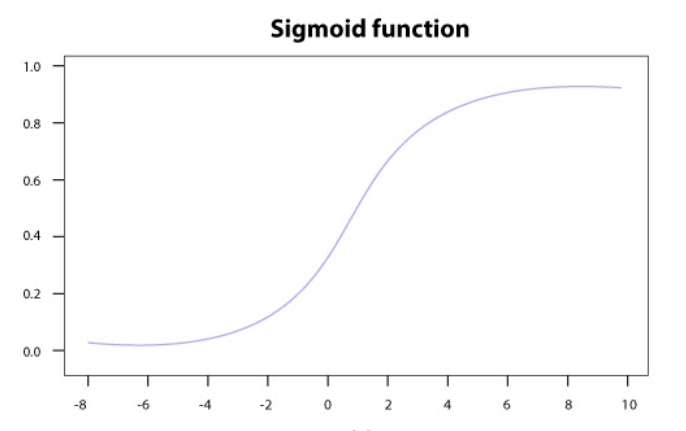#### Logistic Regression in Machine LearningAnalytics Vidhya

Logistic Regression is a technique that can be used for traditional statistics as well as machine learning. Logistic Regression is similar to Linear Regression except Logistic Regression predicts…#### Introduction to Logistic RegressionAnalytics Vidhya

Have you ever wondered why some people are extended loans or credit cards by banks while some others are not? How do banks know with some amount of surety that the individuals to whom they provide…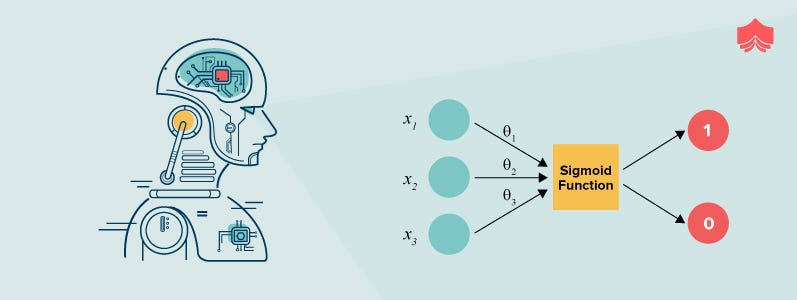#### Logistic Regression | Detailed ExplanationAnalytics Vidhya

Logistic Regression is a statistical method used for classification by measuring the relationship between categorical dependent variable and independent variable by using logistic function. However …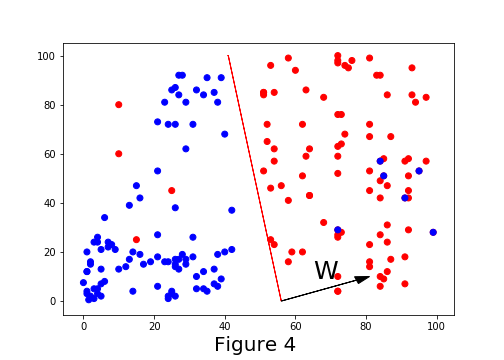#### Logistic Regression Part-IAnalytics Vidhya

There are many ways for understanding Logistic Regression. In this article, we shall learn with a graphical(Geometrical intuition) way of representing Logistic Regression. Even though the name itself…...#### LOGISTIC REGRESSION CLASSIFIERTowards Data Science

With this second post of series, we will continue from ‘Optimizing Objectives’ subject and try to finish all remaining topics listed in below table. where s is the number of successful (Head)…

Read more at Towards Data Science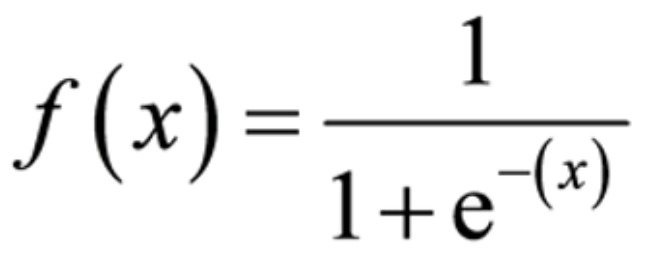#### Machine Learning-Logistic RegressionAnalytics Vidhya

Logistic Regression aka classification is a subset of Supervised learning. Classification machine learning models depends on the binary output. One such model is the sigmoid function. The main reason…...